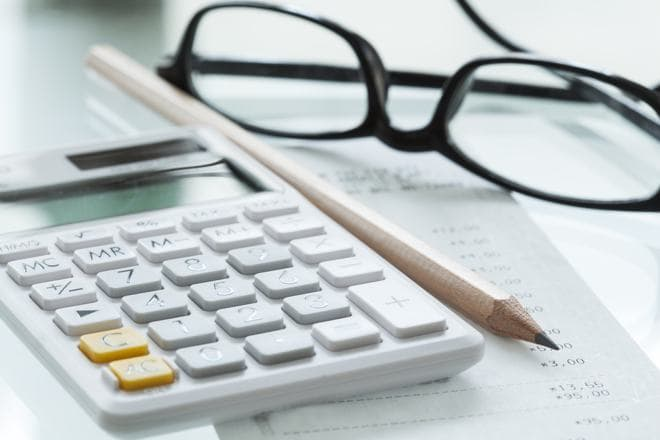Thursday, September 28

# How Compound Interest Works and How to Calculate It

0
528Lending or borrowing money is charged at an interest. The borrower must pay this charge to the lender as the interest is levied on renting money. The profits of lending money are earned at an interest rate. Interest rate is the percentage at which the borrower must pay the charge, and compound interest is one form of interest.

Compound interest is a charge levied on interest earned and principal amount. The interest earned on principal amount in one term is added to the next term. Only this time, the interest is levied on the total amount and not only the principal amount. When a person lends money at compound interest, they can earn more profits as there is an increase in the principal amount every term.

How It Works

For example-

You invest Rs. 1000 in a fixed deposit account for 4 years at an interest rate of 10% per annum. The principal amount in the first term is Rs. 1000. You earn Rs. 100 after the term as the interest is charged at 10%. However, in the next term, your principal amount will not be Rs. 1000, it will be Rs. 1,100. You will earn Rs. 110 in the second year at an interest rate of 10%. The principal amount has now increased to Rs. 1,210. In the next term, you will earn Rs. 121. The total amount is now Rs. 1,331. After that term, you will earn Rs. 133.10 at an interest rate of 10%. The principal amount increases to Rs. 1,464.10. The fixed deposit will have earned you a profit of Rs. 464.10 in 4 years and it is due to compound interest.

Compound interest is levied every term on the principal amount and the interest earned from a term. The lender can make high profits with compound interest. Normally, there are two important factors that decide the interest rate, inflation and credibility of the borrower. The returns from compound interest are impacted by its terms. Also, interest rate is an important factor.

The different terms of compound interest are-

• Annually (Compound interest levied every year)
• Quarterly (Compound interest levied every 4 months)
• Weekly (Compound interest levied 52 weeks in a year)
• Daily (Compound interest levied 365 days)

Compound Interest Factors

• Term

It is important that a person lends money for a longer time. Only then can they earn higher profits. Compound interest will be calculated more times if the term is long. Also, the number of terms is important. If the terms are more, then the returns can be more.

• Interest Rate

Interest rate is the percentage at which the principal amount is charged. A higher interest rate can earn more profits.

• Principal Amount

The first amount that a person lends is the principal amount. However, the principal amount consistently increases when it is compounded. While the principal amount is important, the term and the interest rate have a higher impact.

P = Principal Amount

R = Interest Rate (in decimals)

N = Number of Compounding Terms

T = Total Time

A = Amount After Terms

How to calculate compound interest-

A = P(1+R/N)NT

People earning at a compounded rate of interest earn higher returns. The lender can increase their amount a lot by investing in instruments that offer compound interest.